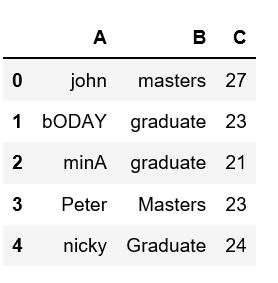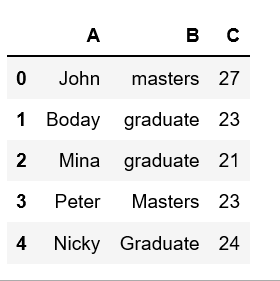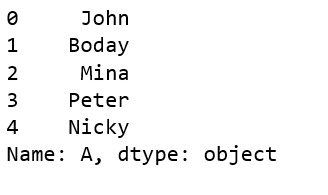# Capitalize first letter of a column in Pandas dataframe

Analyzing real-world data is somewhat difficult because we need to take various things into consideration. Apart from getting the useful data from large datasets, keeping data in required format is also very important.

One might encounter a situation where we need to capitalize any specific column in given dataframe. Let’s see how can we capitalize first letter of a column in Pandas dataframe.

Let’s create a dataframe from the dict of lists.

 `# Create a simple dataframe ` `  `  `# importing pandas as pd ` `import` `pandas as pd ` ` `  `  `  `# creating a dataframe ` `df ``=` `pd.DataFrame({``'A'``: [``'john'``, ``'bODAY'``, ``'minA'``, ``'Peter'``, ``'nicky'``], ` `                  ``'B'``: [``'masters'``, ``'graduate'``, ``'graduate'``, ` `                                   ``'Masters'``, ``'Graduate'``], ` `                  ``'C'``: [``27``, ``23``, ``21``, ``23``, ``24``]}) ` `  `  `df `

Output:There are certain methods we can change/modify the case of column in pandas dataframe. Let’s see how can we capitalize first letter of columns using `capitalize()` method.

Method #1:

 `# Create a simple dataframe ` `  `  `# importing pandas as pd ` `import` `pandas as pd ` ` `  `  `  `# creating a dataframe ` `df ``=` `pd.DataFrame({``'A'``: [``'john'``, ``'bODAY'``, ``'minA'``, ``'Peter'``, ``'nicky'``], ` `                  ``'B'``: [``'masters'``, ``'graduate'``, ``'graduate'``, ` `                                   ``'Masters'``, ``'Graduate'``], ` `                  ``'C'``: [``27``, ``23``, ``21``, ``23``, ``24``]}) ` `  `  `df[``'A'``] ``=` `df[``'A'``].``str``.capitalize() ` ` `  `df `

Output:Method #2: Using lambda with capitalize() method

 `# Create a simple dataframe ` `  `  `# importing pandas as pd ` `import` `pandas as pd ` ` `  `  `  `# creating a dataframe ` `df ``=` `pd.DataFrame({``'A'``: [``'john'``, ``'bODAY'``, ``'minA'``, ``'Peter'``, ``'nicky'``], ` `                  ``'B'``: [``'masters'``, ``'graduate'``, ``'graduate'``, ` `                                   ``'Masters'``, ``'Graduate'``], ` `                  ``'C'``: [``27``, ``23``, ``21``, ``23``, ``24``]}) ` `  `  `df[``'A'``].``apply``(``lambda` `x: x.capitalize()) `

Output:Attention geek! Strengthen your foundations with the Python Programming Foundation Course and learn the basics.

To begin with, your interview preparations Enhance your Data Structures concepts with the Python DS Course.

My Personal Notes arrow_drop_upCheck out this Author's contributed articles.

If you like GeeksforGeeks and would like to contribute, you can also write an article using contribute.geeksforgeeks.org or mail your article to contribute@geeksforgeeks.org. See your article appearing on the GeeksforGeeks main page and help other Geeks.

Please Improve this article if you find anything incorrect by clicking on the "Improve Article" button below.

Article Tags :

Be the First to upvote.

Please write to us at contribute@geeksforgeeks.org to report any issue with the above content.